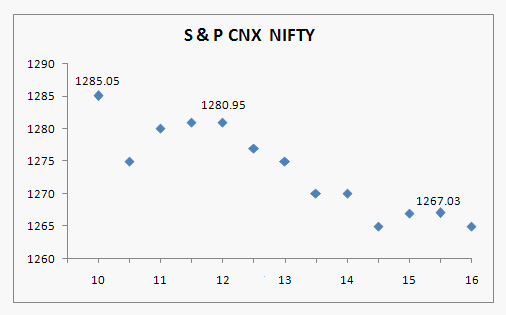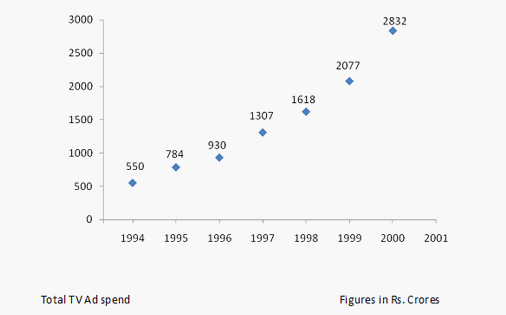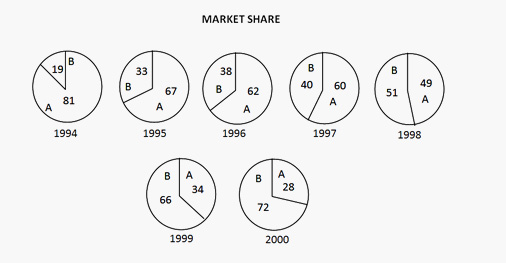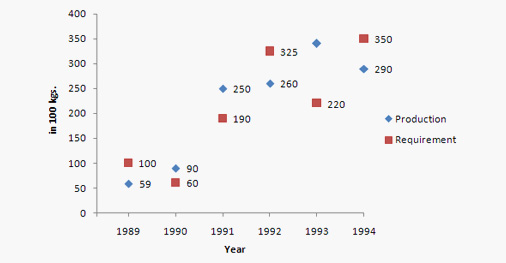# Scattered Plot Practice Questions : Level 02

DIRECTIONS for questions 1 &2: The following graph gives the movement of the stock market index, CNX Nifty over one week.1. If I had invested Rs 100 in S&P CNX Nifty stocks at the beginning of the time period, what would be its nearest value at the end of the period?
1. Rs 99.50
2. Rs 98.50
3. Rs 97.50
4. Rs 96.50
2. Price volatility (PV) is defined as: [(Highest - Lowest Price) / Average Price]. The PV of S&P CNX Nifty is closest to-
1. 0.10
2. 0.001
3. 0.01
4. 1.0
DIRECTIONS for questions 3 to 6: Refer to the graph given below which shows the total advertisement spending on television and the pie chart which  gives the percentage share of the revenue of different players and answer the questions that follow:A denotes DD share. B denotes C&S share. Figures in percentages.
1. What is the percentage increase of revenues of C & S from 1995 to 1996?
1. 43.2 %
2. 57.4 %
3. 15.1 %
4. 36.6 %
2. The market share of C & S has:
1. First increased, then decreased
2. Increased at a steady rate
3. Increased at a faster rate initially, then declined
4. None of these
3. The compounded annual growth rate (of the total TV advt. spending) from 1995-1999 would be closest to:
1. 27 %
2. 15 %
3. 42 %
4. 66 %
4. If in 2001 the growth rate of C&S was same as that of 1999-2000, the total market share of C&S in 2001 would be:
1. 75 %
2. 76.7 %
3. 82 %
4. Can't be determined
Directions for question 7 to 10: Refer to the following questions  which are based on the graph given below:
Requirement and Production of flowers over the years1. The total of requirements in the year 1991 and 1992 is approximately what percent more than the total of production for these two years?
1. 0.098%
2. 9.8%
3. 0.98%
4. None of these
2. During which year was the percentage increase in the requirement of flowers over the previous year, the maximum?
1. 1993
2. 1992
3. 1991
4. 1994
3. If the production in 1995 were to decrease at the same rate percent as it decreased from 1993 to 1994, then what would have been the production in 1995(approx.)?
1. 230
2. 220
3. 225
4. 246
4. For which two years was the average annual production of flowers equal to the average annual requirements?
1. 1991 & 1994
2. 1992 & 1993
3. 1991 & 1992
4. 1993 & 1994